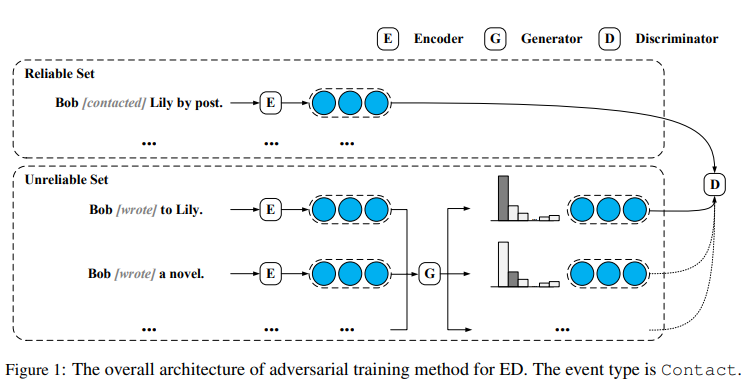# 【论文笔记】Adversarial Training for Weakly Supervised Event Detection

## 简介

1. 事件检测
事件检测（ED）的目的是检测事件触发器（通常是在实例中引发事件的单词或短语），然后识别它们的特定事件类型。
• 特征工程，如token级特征和结构化特征。
• 神经网络模型，将文本语义信息直接嵌入到低维空间中，基于这些特征向量检测事件触发器，采用有监督学习的方法对人类标注数据进行模型训练。

1. 弱监督学习
参考资料 https://baijiahao.baidu.com/s?id=1594091883249224246&wfr=spider&for=pc
目前广泛采用的弱监督方法充分利用了大量的原始数据，特别是一些具体的信息提取工作，探索了弱监督自动标注ED训练数据的方法，这种弱监督方法可以有效地推广到实际的ED应用中，而不需要大量的劳动。
弱监督方法虽然取得了很好的结果，但仍然存在一些严重的问题：
• 会受到数据中不可避免的噪声的影响
• 现有的弱监督ED模型采用复杂的预定义规则和不完全的知识库来自动获取数据，导致数据的自动标注覆盖率低、主题偏差大
1. 本文提出的模型
为了构建一个覆盖范围更广的大规模数据集，减少主题偏差
避免采用复杂的预定义规则和繁重的语义组件分析工具包，提出了一个简单的基于触发器的潜在实例发现策略
一个假设：如果一个给定的单词作为已知事件实例中的触发器，那么提到这个单词的所有实例也可以表示一个事件
与复杂的规则相比，该策略在单词、触发器和事件类型之间的相关性方面限制较少。因此，我们的策略可以在不需要任何人工设计的情况下，获得覆盖更多主题和实例的候选集。

• 将数据集分为可靠集和不可靠集
• 设计了一个鉴别器（判断给定实例的信息量和注释是否正确）和一个生成器（从原始数据中选择最易混淆的实例来欺骗鉴别器）
• 以可靠数据为正实例，以生成器选择的数据为负实例，对判别器进行训练
• 训练生成器选择数据以欺骗鉴别器
• 在训练过程中，生成器可以提供大量的潜在噪声数据来增强鉴别器，鉴别器可以影响生成器选择那些信息量更大的数据
• 由于噪声数据对生成器和鉴别器的优化都没有影响，本文将短语视为单词
• 当生成器和鉴别器达到平衡时，鉴别器可以提高对噪声的抵抗能力，更好地对事件进行分类，生成器可以有效地为鉴别器选择信息实例
1. 本文提出的实验
在半监督和远程监督两种情况下进行了实验。
实验结果表明，基于触发器的潜在实例发现策略对抗性训练方法能够协同获得更为多样化和精确的训练数据，并减少噪声问题的副作用，从而显著优于现有的ED模型。

### 相关工作

• 基于特征的方法。依赖于人工设计的特征来检测事件触发器和事件类型。
• 神经网络方法
• 利用外部知识、上下文信息、文档级信息和多模式集成来提高ED系统的性能
• 先进的体系结构，如注意机制、图卷积网络和生成对抗网络

• 无监督方法和各种弱监督方法。
• 采用远程监控的方式为低资源语言创建训练实例
• 采用WordNet和基于规则的方法生成没有事件类型标签的开放域数据
• 在知识库中利用远程监控从现有结构化事件知识中生成大规模数据
• 用自举进行半监督学习

### 模型

#### 框架##### 实例编码器

CNN 在将实例$x$中的所有单词表示为它们的输入嵌入（包括单词嵌入和位置嵌入,它们对候选触发器的相对位置进行编码）后，CNN在输入嵌入上滑动卷积核以获得隐藏嵌入

$\{h_1,...,h_n\}=CNN(w_1,...,t,...,w_n)$

BERT 类似于CNN，在将实例$x$中所有单词的词条、段和位置嵌入相加作为输入嵌入后，BERT采用多层双向变压器编码器得到隐藏嵌入

$\{h_1,...,h_n\}=BERT(w_1,...,t,...,w_n)$

$[x_l]_j = max\{[h_1]_j ,..., [h_i ]_j\}\\ [x_r]_j = max\{[h_{i+1}]_j,...,[h_n]_j\}\\ x=[x_l;x_r]$

##### 对抗策略

• 鉴别器检测数据集中每个实例的事件触发器和事件类型，当给定一个有噪声的实例时，鉴别器也要抵抗噪声，并明确指出没有触发器和事件。
• 生成器用于从不可靠的数据集$\mathcal{U}$中选择实例，以尽可能地混淆鉴别器。

$\phi _D=max(E_{x∼P_R}[logP(e|x,t)]+E_{x∼P_U}[log(1 − P(e|x, t))\\ \phi _G=max(E_{x∼P_U}[logP(e|x,t)])$

##### 鉴别器

$D(e|x,t)=e·x\\ P(e|x,t)=\frac{exp(D(e|x,t))}{\sum_{\hat{e}∈\mathcal{E}}exp(D(\hat{e}|x,t)}$

$\mathcal{L}_D=−\sum_{x∈\mathcal{R}}\frac{1}{|R|}logP(e|x,t)−\sum_{x∈\mathcal{U}}P_{\mathcal{U}(x)}log(1−P(e|x,t))$

##### 生成器

$f(x)=W·x+b\\ P_\mathcal{U}(x)=\frac{exp(f(x))}{P_{\hat{x}∈\mathcal{U}}exp(f(\hat{x}))}$

$\mathcal{L}_G=−\sum_{x∈\mathcal{U}}P_{\mathcal{U}}(x)logP(e|x,t)$

$P(NA|x,t)=\frac{1}{|\mathcal{E}|−1}\sum_{e∈\mathcal{E},e\neq NA}P(e|x,t)$

##### 训练和应用细节

$\widetilde{\mathcal{L}}_D = −\sum_{x∈\widetilde{\mathcal{R}}}\frac{1}{|\widetilde{\mathcal{R}}|}logP(e|x,t)−\sum_{x∈\widetilde{\mathcal{U}}}P_{\widetilde{\mathcal{U}}}(x) log(1−P(e|x,t))\\ \widetilde{\mathcal{L}}_G = −\sum_{x∈\widetilde{\mathcal{U}}}P_\widetilde{\mathcal{U}}(x)log(P(e|x,t))$

$P_{\tilde{\mathcal{U}}}(x)=\frac{exp(f(x)^\alpha)}{P_{\hat{x}∈\tilde{\mathcal{U}}}exp(f(\hat{x})^\alpha)}$

α是一个超参数，它控制概率分布的锐度，以避免权重集中在某些特定实例上

$\mathcal{L}=\widetilde{\mathcal{L}}_D+\lambda\widetilde{\mathcal{L}}_G$

$\widetilde{\mathcal{L}}_G$的学习率中加入了λ，避免了对λ的额外调整

##### 适应弱监督场景

• 基于触发器的潜在实例发现：利用未标记的数据

• 半监督场景下采用对抗性训练策略

• 远程监控场景下采用对抗性训练策略

posted @ 2019-11-27 17:48  "kisetsu  阅读(767)  评论(0编辑  收藏  举报